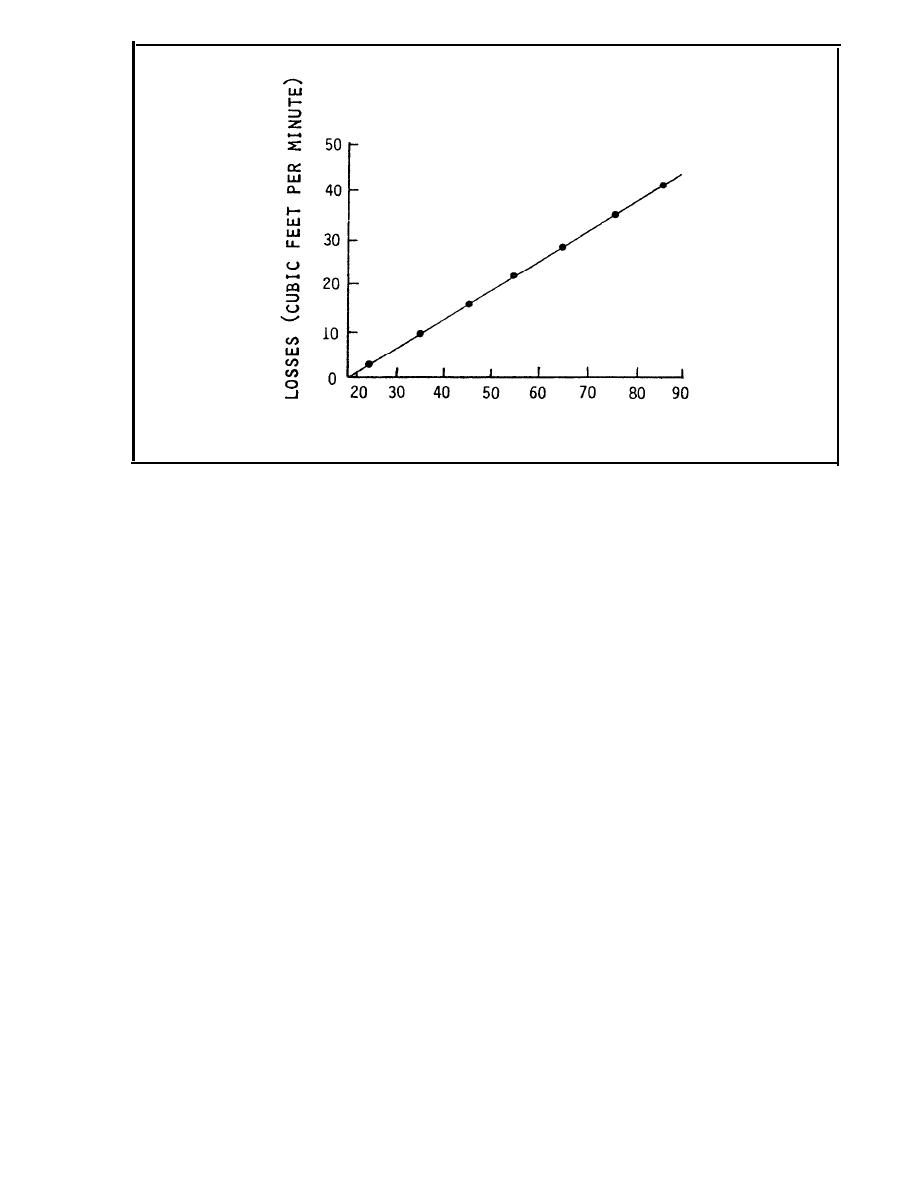Figure B-1. Loss (cfm) vs Pressure (psig)Custom SearchAVERAGE PRESSURE (PSIG)
FIGURE B-l. Loss (cfm) vs Pressure (psig)
(f) Calculating the linear regression formula from the available data
and making the following substitutions:
X
= avg press. (P)
Y
= loss (Q)
Y
= mX+b
yields the equation: Q = 0.62 P - 12.75.
Losses at operating or nominal pressure (96 psig) are calculated:
Q  9 6 = 47 scfm
Q9 6  = 0.62 (96) - 12.75
At a cost of 50 cents for 1,000 cubic feet of compressed air,
this represents: \$12,35O/year (dollar figure will vary based on
facility costs for compressed air).
6 CORRECTIVE MEASURES. Qnominal represents the loss in the compressed air
system at operating conditions, assuming a constant pressure over the length
of pipe in question. This value, taken with the activity's cost to produce
compressed air, can be used, as justification,to develop projects to repair
or replace sections of compressed air line.
B-6Integrated Publishing, Inc.